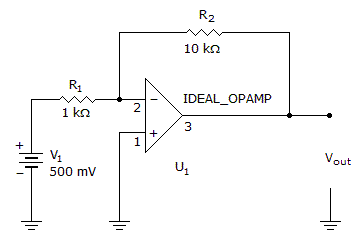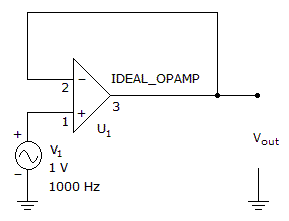# Electronics - Operational Amplifiers

### Exercise :: Operational Amplifiers - General Questions

21.

All of the following are basic op-amp input modes of operation EXCEPT

 A. inverting mode B. common-mode C. double-ended D. single-ended

Explanation:

No answer description available for this question. Let us discuss.

22.

What is the output voltage?A. 15 V B. 5 V C. –5 V D. –15 V

Explanation:

No answer description available for this question. Let us discuss.

23.

A circuit whose output is proportional to the difference between the input signals is considered to be which type of amplifier?

 A. common-mode B. darlington C. differential D. operational

Explanation:

No answer description available for this question. Let us discuss.

24.

With negative feedback, the returning signal

 A. is proportional to the output current B. is proportional to the differential voltage gain C. opposes the input signal D. aids the input signal

Explanation:

No answer description available for this question. Let us discuss.

25.

What is the output waveform?A. sine wave B. square wave C. +15 V D. –15 V